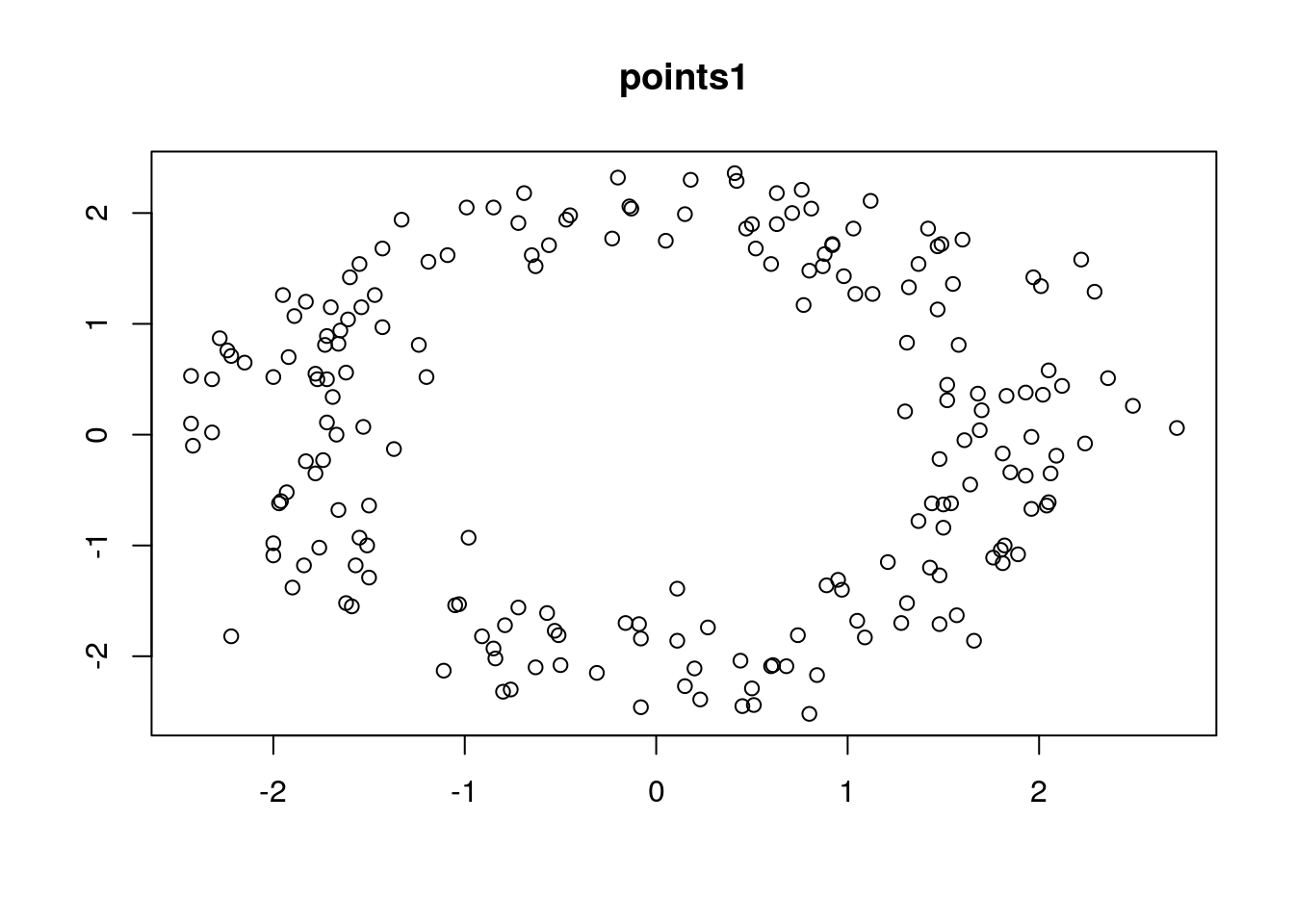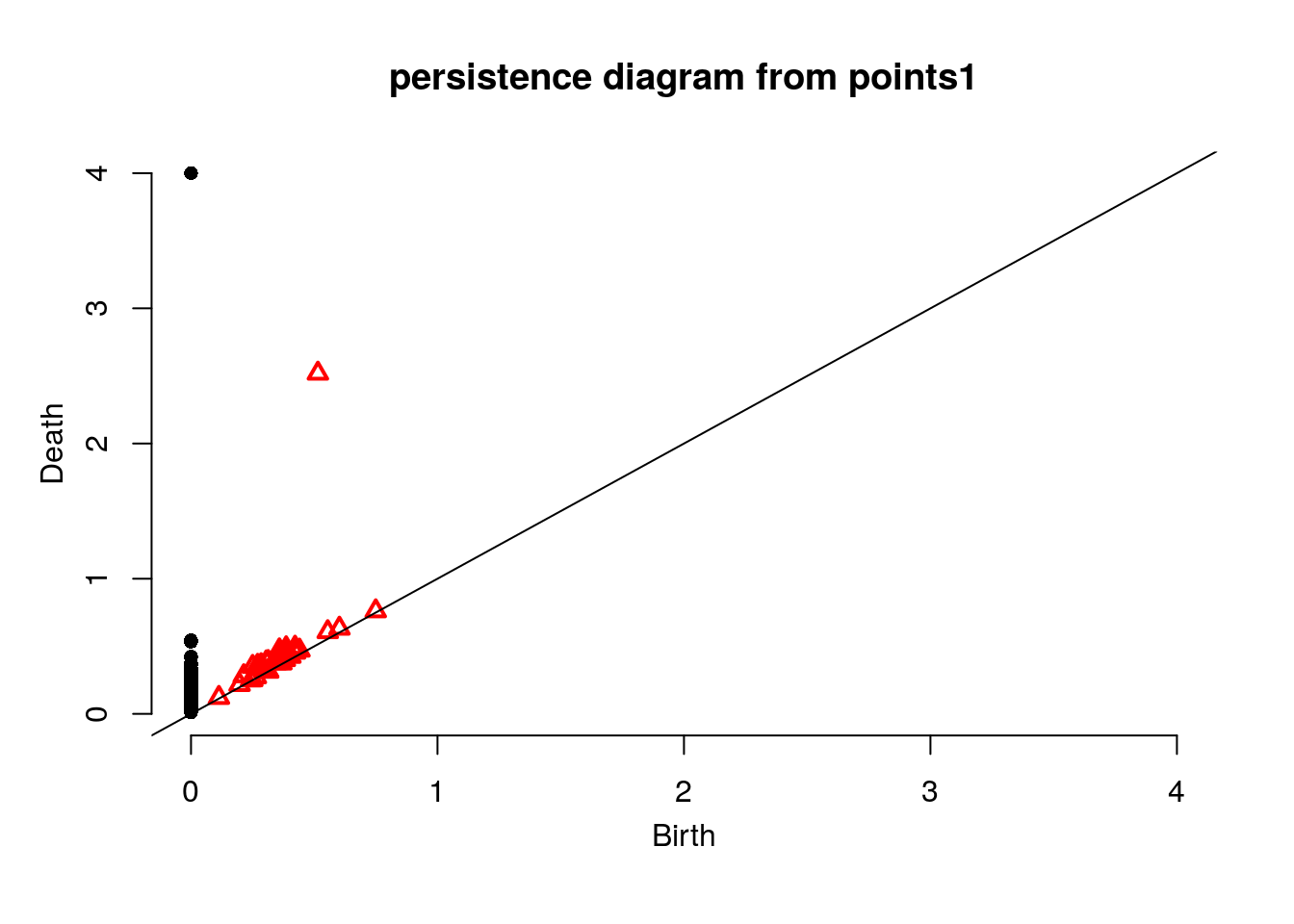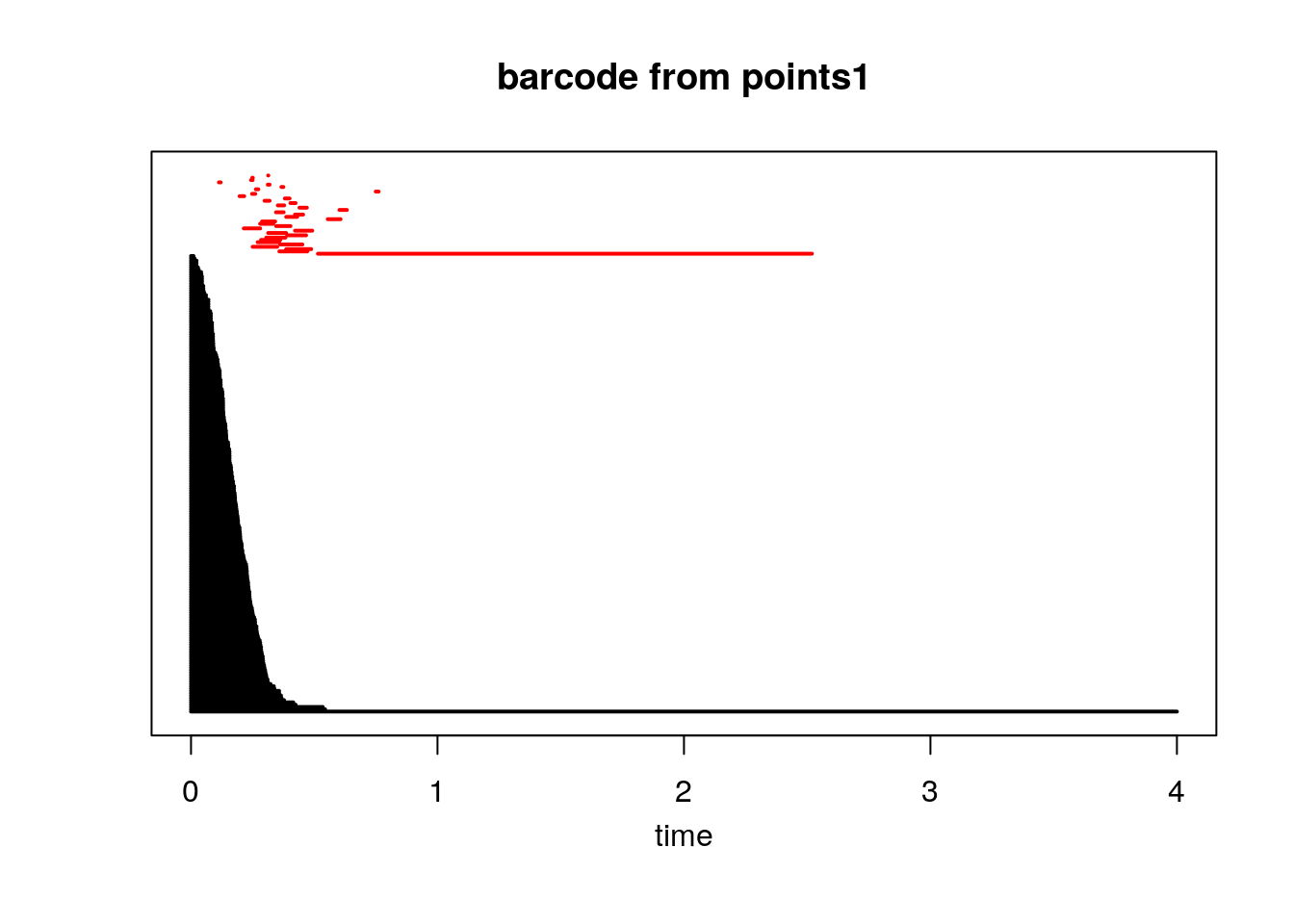Note that a more detailed tutorial on the R TDA package is available here.

Before we begin, load the TDA package. (If the package is not installed, you can install it using RStudio, or type `install.packages("TDA")`.)

``library("TDA")``

## Vietoris-Rips Persistence

The `ripsDiag` function computes the persistence diagram of a Vietoris-Rips complex. It returns a list containing a diagram object, which can be plotted either as a persistence diagram or as a barcode. The `ripsDiag` function can use any of the libraries GUDHI, Dionysus, or PHAT.

``````points1 <- read.csv("~/Dropbox/data/point cloud scripts/points1.csv")
plot(points1, xlab="", ylab="", main="points1")````````````Diag1 <- ripsDiag(X = points1, maxdimension = 1, maxscale = 4, library="GUDHI", location = FALSE, printProgress = FALSE)
plot(Diag1[["diagram"]], main="persistence diagram from points1")````````plot(Diag1[["diagram"]], barcode=TRUE, main="barcode from points1")``## Superlevelset Persistence

We first compute a density estimator for the point cloud. We will use the Gaussian Kernel Density Estimator (KDE), computed over a grid of points in the plane. We first set up the grid, then compute the density estimator using the `kde` function provided by the TDA package. We can also plot the density estimator using the perspective plot function `persp`. Note that the `persp` function requires many parameters to set the viewing direction and shading.

``````Xlim <- c(-3,3)
Ylim <- c(-3,3)
by <- 0.1
Xseq <-seq(Xlim, Xlim, by=by)
Yseq <-seq(Ylim, Ylim, by=by)
Grid <- expand.grid(Xseq, Yseq)
points1kde <- kde(X=points1, Grid = Grid, h = 0.3)
persp(Xseq, Yseq, matrix(points1kde, ncol = length(Yseq), nrow = length(Xseq)), xlab = "",ylab = "", zlab = "", theta = -20, phi = 35, ltheta = 50, col = 2, d = 0.5, main="KDE points1")``````